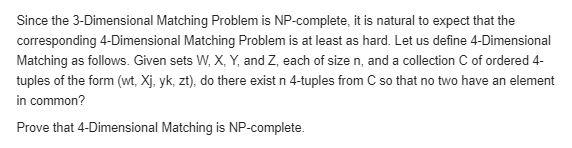# Question Since the 3-Dimensional Matching Problem is NP-complete, it is natural to expect that the corresponding 4-Dimensional Matching Problem is at least as hard. Let us define 4-Dimensional Matching as follows. Given sets W, X, Y, and Z, each of size n, and a collection C of ordered 4- tuples of the form (wt, Xj, yk, zt), do there exist n 4-tuples from C so that no two have an element in common? Prove that 4-Dimensional Matching is NP-completeWNVF4C The Asker · Computer ScienceTranscribed Image Text: Since the 3-Dimensional Matching Problem is NP-complete, it is natural to expect that the corresponding 4-Dimensional Matching Problem is at least as hard. Let us define 4-Dimensional Matching as follows. Given sets W, X, Y, and Z, each of size n, and a collection C of ordered 4- tuples of the form (wt, Xj, yk, zt), do there exist n 4-tuples from C so that no two have an element in common? Prove that 4-Dimensional Matching is NP-complete
More
Transcribed Image Text: Since the 3-Dimensional Matching Problem is NP-complete, it is natural to expect that the corresponding 4-Dimensional Matching Problem is at least as hard. Let us define 4-Dimensional Matching as follows. Given sets W, X, Y, and Z, each of size n, and a collection C of ordered 4- tuples of the form (wt, Xj, yk, zt), do there exist n 4-tuples from C so that no two have an element in common? Prove that 4-Dimensional Matching is NP-complete